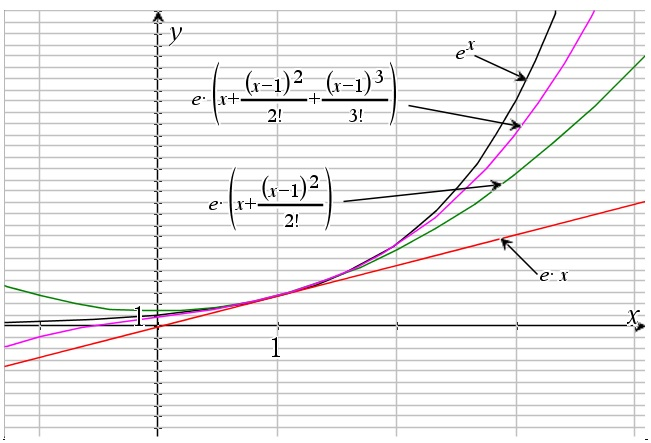# Taylor series

Up a level : Power Series
Previous page : Mclaurin series - a first take
Next page : Integrating and differentiating a seriesTaylor series are basically Mclaurin series of a function horizontally translated by a units – or Mclaurin series are Taylor series without any translation. We have$f(x) = \sum\limits_{k = 0}^\infty {\frac{{{f^{(k)}}(a)}}{{k!}}{{(x - a)}^k}}$

This may be used if we want to have a series expansion that is converging quickly when x is close to some other value than 0.  Let us for example look at ex again, but now cantered about 1. So we get$\begin{gathered} {e^x} = \sum\limits_{k = 0}^\infty {\frac{{{e^1}}}{{k!}}{{(x - 1)}^k}} = e\sum\limits_{k = 0}^\infty {\frac{{{{(x - 1)}^k}}}{{k!}}} \hfill \\ \quad = e\left( {1 + (x - 1) + \frac{{{{(x - 1)}^2}}}{{2!}} + \frac{{{{(x - 1)}^3}}}{{3!}} + ...} \right) \hfill \\ \quad = e\left( {x + \frac{{{{(x - 1)}^2}}}{{2!}} + \frac{{{{(x - 1)}^3}}}{{3!}} + ...} \right) \hfill \\ \end{gathered}$

A graph of this is seen below for the first few terms of the expansion.Up a level : Power Series
Previous page : Mclaurin series - a first take
Next page : Integrating and differentiating a seriesLast modified: Mar 15, 2019 @ 09:12